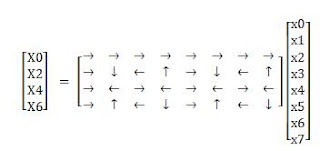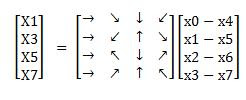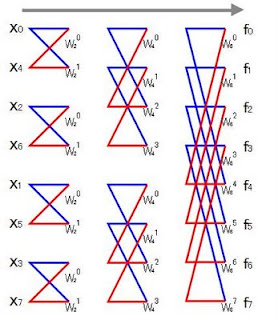## 2011年8月31日水曜日

### SRM 516 (div2) Exercise

250がいつもよりちょっと難しめ、500は普通、1000はいつもより簡単に感じた。

250 NetworkXZeroOne
'o'と'x'からのみなる文字列を送信する。ネットワークの不調のため文字列のいくつかの部分が壊れてしまい、受信側では、"o?x?"や"????x"のような状態になっている。この文字列を復元する。ただし、文字列のすべての偶数部分列に含まれる'o'と'x'の数は等しい。

少なくとも一つの'?'の前、もしくは後ろは、'o'か'x'になっているはず。なので、それとは違う文字を'?'に入れてやればいい。これを'?'が無くなるまで繰り返す。今回はサイズが小さいので愚直に文字全体をなめていっても間に合う。サイズが大きい場合は、queueをつかって、新しくfixした部分の周りだけ見るという工夫(priority_queueを使ってdijkstraを高速化するときと同じようなやり方)をすると高速化できる。

バイナリの平文（'0'と'1'からのみ構成される平文）を、あるキー文字列を使って暗号化する。

要するに、暗号化を写像fに見立てた場合、fがonto(surjective)であればいい。

1000 NetworkXMessageRecovery
アルファベットの大文字、小文字のみからなる文字列xがある。ただし、この文字列のなかに同じ文字は2回以上現れない。xの部分文字列（必ずしも連続していない）が複数個与えられるので、xが何かを予想する。xが複数個考えられる場合は、辞書順がもっとも小さいものを出力する。

 {"acd", "bce"}　-> "abcde"
{"ed", "dc", "cb", "ba"} -> "edcba"

それぞれの部分列から、どの文字がどの文字より先に現れるべきかが分かる。ある文字aが別の文字bに先行する場合は、aからbへの有向枝があると考えてグラフを構成する。これをトポロジカルソートしてあげればいい。ただしトポロジカル順序の決定は辞書順が小さいものから行うようにする。O(n^3)。

## 2011年8月28日日曜日

### A few Tips for Multidimensional Arrays in C/C++

A couple of questions about multidimensional arrays has occurred to my mind.
1. memory set of multidimensional arrays
2. abstract method of dealing indices corresponding to dimensions
The first one is simple. I got a little confused when I was doing something like this:

int dp;int main() {    /*     * some procedures here     */    memset(dp, 0, sizeof(dp));}

So you want to set dp[i][j], i=0,1,..,63, j = 0,1,..,63, to zeros. Does this really work? what is the size of dp?? A multidimensional array are actually a One-dimensional array. More specifically, memories are allocated to a multidimensional array as if it were a one-dimensional array. So dp represents the address of dp. So the size is 16384byte(64*64*8byte). It makes sense.

But how's about when you want to reset dp[i][j], i=0,1,..,63, j=0,1,..,63, leaving dp[i][j] unchanged? Does memset(dp, 0, sizeof(dp)) work? It's confusing this time.
What is the size of dp? 16384byte? or 32768byte? The former is correct. Which reminds me that I sometimes do this kind of thing:

int buf;int main() {    cout << sizeof(buf) / sizeof(buf) << endl;         // 8    cout << sizeof(buf) / sizeof(buf) << endl;   // 16}

The second question is difficult to describe. So I've prepared a simple, original problem to get the essence.

You are given a 6-dimensional array A. Each element of A has an integer. You are given an integer n, an integer between 0 and 5, inclusive, which is the index of a moving dimension, and six integers (d0, d1, d2, d3, d4, d5), all of whom are integers between 0 and 7, inclusive. Calculate the sum A[d0'][d1'][d2'][d3'][d4'][d5'], where di' = {0,1,2,...,7} if i =n, otherwise di. (Sorry this statement may be awkward. In that case, see the example below.)

Here's an example. n = 1, (d1, d2, d3, d4, d5, d6) = (0,1,2,3,4,5).
You are to calculate A + A + A + A + A + A + A.

I first wrote a source code like this:

int A;void init() {    REP(d0, 8)REP(d1, 8)REP(d2, 8)REP(d3, 8)REP(d4, 8)REP(d5, 8)        A[d0][d1][d2][d3][d4][d5] = d0 + d1 + d2 + d3 + d4 + d5;}int main() {    int n;    int d0, d1, d2, d3, d4, d5;    init();    cin >> n;    cin >> d0 >> d1 >> d2 >> d3 >> d4 >> d5;    int ans = 0;    switch (n) {    case 0:        REP(i, 8) ans += A[i][d1][d2][d3][d4][d5];        break;    case 1:        REP(i, 8) ans += A[d0][i][d2][d3][d4][d5];        break;    case 2:        REP(i, 8) ans += A[d0][d1][i][d3][d4][d5];        break;    case 3:        REP(i, 8) ans += A[d0][d1][d2][i][d4][d5];        break;    case 4:        REP(i, 8) ans += A[d0][d1][d2][d3][i][d5];        break;    default:        REP(i, 8) ans += A[d0][d1][d2][d3][d4][i];    }    cout << ans << endl;}

Talk about a terrible code. Once you notice that a address is linear, you can write this cool code -- a friend of mine taught this implementation --. In this case, the size of each dimension is 8, imagine the base 8 number system, which will help you understand the code.

int A;void init() {    REP(d0, 8)REP(d1, 8)REP(d2, 8)REP(d3, 8)REP(d4, 8)REP(d5, 8)        A[d0][d1][d2][d3][d4][d5] = d0 + d1 + d2 + d3 + d4 + d5;}int main() {    int n;    int d;    init();    cin >> n;    REP(i, 6) cin >> d[i];    d[n] = 0;    int ans = 0;    int *p = (int *)A;    int base = 1, move = 0;    REP(i, 6) {        if (6-1-i == n)            move = base;        p += d[6-1-i] * base;        base *= 8;    }    REP(i, 8)        ans += *(p + move * i);    cout << ans << endl;}

## 2011年8月27日土曜日

### Probability Theory Practices (Medium 1)

Div1. medium 5問解いた。昔のSRMは比較的簡単なようで、なんとか自力で解けた。
level 1の問題は簡単だったけど、この辺のレベルになると、なかなか面白い問題が集まっている。

確率の問題をパターン化してストックしたいので、方針・実装方法とかをまとめておく。

Collision - SRM 153
分散システム（N個のcomponentから構成される）を用いて、各端末に固有IDを割り振る。分散システム同士は通信を行わない。それぞれのcomponentは、assignments[i]個のIDを端末に割り振らないといけない。以下2つの方針で実装した場合のIDの衝突確率を求める。
1. 各componentはmemoryを持っており、自身が割り当てたIDを記憶できる。よって自身のタスクではcollisionは発生しない。
2. 各componentはmemoryを持っていない。自身のタスクでもcollisionが発生しうる。
2.は使っていないIDを選んでいくだけ。分子がデクリメントされていくような分数の積。1.はcomponentの中では、衝突が起きないように人為的な選択ができるので、（同一component内では）分母もデクリメントされていく。結果、1.の方がcollisionが起きにくくなる。この問題は、数学色が強い。実装は簡単。

ChessKnight - TCCC05 Round 1
8*8のチェス盤上に一駒のknightが任意の場所に置かれている。knightは一様な確率で8方向（通常のknightの動きと同じ）のいずれかにランダムに移動する。knightをN回移動させたときに、knightがチェス盤上に残っている確率を求める。

ぱっと見、むずかしそうだなと思った。が、DPを使えば、i回移動させたとき各マス上にknightがいる確率が分かれば、(i+1)回目移動させたときにあるマス上にknightがいる確率は計算できる。O(8)。これをN回、すべてのマス上で行えばよいので、O(8*8*8*N)で計算できる。

ChipRace - SRM 199
ポーカーで賭けをするとき、レートが5倍にあがる。というときがある。そのときN人のプレイヤーの手持ちがそれぞれ5の倍数なら問題ないが、端数がでた場合は、chip raceという確率的なミニゲームをおこなう。chip raceでは一人最高でも1つのチップしか勝ち取ることはできない。ゲームの状態が与えられるので、各プレイヤーがチップを勝ち取る確率を求める。

一度チップを勝ち取ったプレイヤーは、さらにチップを勝ち取ることはないので、そのプレイヤーは以後のターンでは除外して考えることができる。あるプレイヤーがチップを勝ち取る確率は、
1ターン目で勝つ確率 + 1ターン目で負ける確率 * (2ターン目で勝つ確率 + 2ターン目で負ける確率 * (3ターン目で勝つ確率 + 3ターン目で負ける確率 * (・・・・)))
のような再帰で書ける。また、どのプレイヤーがactiveかをビットで保持することにすれば、状態数M*(1<

実はこの問題、メモ化の機構は不要なことが分かった。最悪ケースは、M=10, N=10程度で、深さ10の10分木ができるかなーというイメージだったが、よくよく考えると、各階層で注目しているプレイヤーが勝つという場合を考えるので、分岐の数は、10, 9, 8, 7・・・と減っていく。なので、10! ~ 3.6*10^6回程度再帰されるだけなので、単なる再帰でもOKだったようだ。ただ、この階乗の形で木をイメージすることが出来ていなかったので、良い勉強になった。DiceThrows - SRM 242
A君と、B君がサイコロをNa回、Nb回ずつ振る。（それぞれ最高で200回）。サイコロは6つ面があり、それぞれの面には、1~ 100までのいずれかのpips（目）が書いている。サイコロを振った合計値を得点とするとき、A君がB君より高い得点を取る確率を求める。ただし、サイコロの各面がでる確率は一様分布に従う。

まずA君の得点がある数字になる確率を部分和問題の要領で計算する。結果は、サイズ20,000程度のバッファdp[]に格納。今度はB君について同じことをする。ただし今度は引き算の方向に処理をする。結果は、dp[i], i=1,2,...の和。

Editorial解はちょっと複雑で、それぞれの得点をdpa[], dpb[]に格納。そのあと、B君の得点がi未満になる確率をpos[i]に格納。dpa[i] * pos[i]の総和を解とする。

相変わらず、部分和問題のときにtmp用のバッファを用意するのがまだまだ成長してないなと思った。下から上じゃなくて、上からしたに見て行けば矛盾なくin-placeで更新できるので、今度からは気を付ける。

TopFive - SRM 243
3つの問題を解いてオンラインジャッジをするコンテストがある。コンテストの参加者は問題を解くのに必要とした時間に応じて点数が与えられる。ただし、ジャッジに落ちた問題の点数は0となる。それぞれの参加者の3つの問題に対する点数と、ジャッジをパスする確率が与えられる。あなたの点数が与えられるので、あなたが上位5位以内に入る確率を求める。

これは、なかなか悩んだ。どこを状態数として保持するか。i人目までをみたときに、あなたより高い点数がj人いる確率をdp[i][j]として保持すると、うまく計算できる。

### Probability Theory Practices (Easy)

確率論の問題を解いた。確率論のtutorial+レベル別問題まとめみたいなのがあったのでやってみた。

level 1:はbrute force系の問題が多かった。

BirthdayOdds - SRM 174
1年がN日から成り立つ世界がある。何人の人を集めれば、同じ誕生日の人が少なくとも2人出てくる確率がP(%)以上になるか？
というような問題。
問題文にあるように、「クラスに23人いれば、同じ誕生日の人がいる確率は50%を超える。」というのは有名な話。counter-intuitiveな確率の話として、覚えておいて損はないかも。

BenfordsLaw - SRM 155
この法則は知らなかった。

これは面白い。数字の最大桁の値は一様分布に従うと思いきや、違うらしい。この法則によると、最大桁が1である確率は、30%を超える。50%の確率で、数字の最大桁の値は、1または2となるらしい。

この問題では、この法則を用いて、金融機関のtransactionの監査を行う。金融機関で取引された金額リストを取得して、すべての数字の最大桁の値dをサンプリングする。適当な閾値を設定しBenford's Lawに従わない値dが存在すれば、それらの取引には何らかの不正があると考え厳重に監視する。

プロコンの問題という観点では単純な実装ゲーだったが、この法則と問題の内容が面白かった。

余談になるが、この問題では予めlog10(1+ 1/d), d=1,2,...,9を計算してstaticなテーブルに入れておくとよい。今回は、求める数値が9つなので起動時に計算しても問題ないが、求める個数が大きくなった場合はメタプログラミングの対象になるのかなと思った。とか何とか考えながら、いろいろ調べてたらこんな変態postを発見。なんだこれは（笑）面白そうじゃなイカ。

## 2011年8月24日水曜日

### 固有値、固有ベクトルの物理的意味を考える

ポイント1この写像を行列を用いて表すと以下のように書くことができる。この写像fを二次元平面上で考えてみる。fは、上図のように黒い点を青い点に写すような写像である。fには、x軸方向のベクトルを2倍にし、y軸方向の値を3倍にするという働きがある。

ポイント2
ベクトル空間の軸の取り方は複数個ある。や、という具合。

実は、既定の取りは複数個存在する。例えば、[2; 1]、[-1; 1]を基底にとってみる。このとき、と２つのベクトルは基底[2;1]、[-1;1]の線形結合で表すことができる。同様に、任意の2次元ベクトルは、これらの基底の線形結合で表すことができる。

さて、[1; 0]、[0; 1]を基底としてグラフを書けば、水平線と、垂直線を軸にとる。[2; 1]、[-1; 1]を基底に選ぶということは、以下のような軸のもとでグラフを書くということを意味している。
ポイント3

以下の行列Aについて考える。この行列は、写像を表現したものだといえる。この写像を基底[1;0]、[0; 1]毎に適応すると、となる。ここで注目することは、上の結果がそれぞれAの列ベクトルと一致しているということである。つまり、基底に対する写像の結果によって行列の形が一意に決まるように見える。となるので、任意のベクトルに対する写像のふるまいは、基底に対するふるまいによって決定される。ここで、基底[0; 1]、[1; 0]をv1、v2に変換するような行列Pを考える。（何の前触れもなくPが登場しましたが、面白いことがわかるのでちょっとだけ我慢してください。）このPに対して、inv(P) * A * Pを計算してみます。9, 0, 4はv1, v2にAを適応したときに出て来ました。これは偶然でしょうか？inv(P) A Pという行列は、
1. 基底を[0;1]、[1;0]から、v1、v2に変換
2. 新しい基底のもとで、線形写像fを適応
3. 基底を[0; 1]、[1; 0]に戻す
という操作に対応しています。（行列を右から左に見て行くことに注意。）3.の操作で[0; 1]、[1; 0]の基底に戻ってきているので、inv(P)A Pの基底[0; 1]、[1; 0]に対する振る舞いを見てみます。これは、v1、v2のもとでのfの振る舞いの式と同じ形（基底にかかる係数部分に注目！）をしています。
つまり、inv(P) A Pという表現は、基底（座標軸）を取りなおした場合に、fはどういう形をしているか？
を表したものになっています。
このように正則行列Pに対して、Aをinv(P) A Pに変換する処理を相似変換といいます。相似変換は座標の取り方を変えただけで、写像そのものは変わっていないことから、変換の前後で以下の値が不変のまま保たれます。
• 行列式
• トレース（主対角成分の和）
• 階数
• 固有値
ポイント4の固有値、固有ベクトルはそれぞれです。Aによって表される写像を、基底[1; -4]、[1; 1]のもとでみてみましょう。基底を[1; 0]、[0; 1]から[1; -4]、[1; 1]に変換する行列Pは、です。相似変換をすると、となります。

n*n行列Aによって表される線形写像fを、固有ベクトルx1, x2, .., xnを基底とした座標系で表現すると、写像fは、x1要素をλ1（x1に対応する固有値）倍、x2要素をλ2倍、....するという簡単な写像に対応している。

つまり、座標の見方を変えるだけで、線形変換の本質的な部分が見えるということですね。

おまけ
このブログを書いてる最中に固有値に関して面白い特性を知ったので今後のためにメモしておく。
1. 固有値の和は、トレースと一致する
2. 固有値の積は、行列式と一致する
この2つは、とても興味深いです。時間があるとき証明でも読んどこうかな。

## 2011年8月23日火曜日

### 行列式の計算

行列式を効率的に計算する方法を考える。

まず、n*n行列Aの行列式は、と定義される。ε()は、与えられた順列が奇順列の場合は-1、偶順列の場合は1となるような関数である。

上の定義より、n*n行列の行列式をそのまま計算すると、O(n*n!)の計算量が必要となってしまう。大きなサイズ(n>20)の行列の行列式を簡単に計算するために、以下の性質を用いる。

const int N = 3;double determinant(const double x[][N]) {    double tmp[N][N];    memcpy(tmp, x, sizeof(tmp));    // make pivots non-zero numbers    for (int i = 0; i < N; i++) {        if (tmp[i][i] != 0)            continue;        int k;        for (k = 0; k < N; k++)            if (tmp[k][i] != 0)                break;        if (k == N)          // all numbers in a column is zero            return 0;        for (int j = 0; j < N; j++)            tmp[i][j] += tmp[k][j];    }    // make a triangular matrix    for (int i = 0; i < N; i++) {        for (int j = 0; j < N; j++) {            if (i == j)                continue;            double c = tmp[j][i] / tmp[i][i];            for (int k = 0; k < N; k++)                tmp[j][k] -=  c * tmp[i][k];        }    }    double det = 1;    for (int i = 0; i < N; i++)        det *= tmp[i][i];    return det;}int main() {    double x[N][N];    for (int i = 0; i < N; i++) {        for (int j = 0; j < N; j++)            x[i][j] = -50 + rand() % 100;    }    cout << determinant(x) << endl;    return 0;}

## 2011年8月21日日曜日

### 固有値・固有ベクトルって何に使うの？

ふと、固有値・固有ベクトルって何がそんなに嬉しいのか？何の役に立つのか？と思っていろいろ調べていた。（対角化してべき乗計算が速くできますだけだと、ちょっと勉強する動機づけとしては弱い。。）そういえば、一年前くらいに読んだpage rankの論文に固有値・固有ベクトルが使われていたのを思い出したので、これをちょこっと紹介。（解釈に間違いなどありましたら、ご指摘ください。）

ここまで、まとめると、
1. リンクをたくさん張られているサイトほど重要とみなす
2. 重要なサイトからのリンクほど、大きな重みを付ける
となります。

あるサイトの重要度を上記の定義にしたがって、定式化してみます。（※本当は、リンク元のページから受け取る重要度をそのページのリンク総数で割るという処理がありますが、簡単のため省略しています。）xは、あなたのページの重要度、xi, i= 1,..,nはそれぞれウェブページiの重要度です。（ウェブページは全部でn個あるとしています。）Ai, i = 1,.., nは、0 or 1の値です。もし、ウェブページiからあなたのページにリンクがあれば1、なければ0です。cは定数で、常に全体のウェブページの重要度の総和が一定となるように正規化しています。

上の式から分かるように、あなたのページの重要度は、他のページの重要度がわかっていないと計算できません。逆に、他のページの重要度も、あなたのページの重要度の影響を受けています。ということで、連立方程式をたてて、すべてのページに適切な重要度を割り当てます。このページでは、実際のpagerankを簡素化して説明しました。実際の処理をもっときちんと知りたいという方は、ラリーページとセルゲイブリンがスタンフォード時代に書いた論文をご参照ください。

### 行列関係用語まとめ

• 単位行列: 対角成分のみが1、残りは0である行列。• スカラー行列: 単位行列をスカラー倍したもの。• 対角行列: 対角成分意外が0である行列。• 正方行列: 行数と列数が等しい行列。
• エルミート行列: 行列Aとその随伴行列A*が等しいとき、Aをエルミート行列とよぶ。随伴行列（共役転置行列ともよばれる）とは、行列に転置をしたあと、各要素をその複素共役に置き換えたもの。例からも分かるように、エルミート行列の対角成分は、実数でないといけない。また、もとの行列のサイズと転置した行列のサイズが同じにならなければならないため、エルミート行列は正方行列である。

• 対称行列: 自身と転置した行列が等しくなうような行列。例からも分かるように、実対称行列は、エルミート行列のsubsetである。

• ユニタリ行列: 逆行列と随伴行列が等しくなる行列。ユニタリ行列は、自身と随伴行列をかけると単位行列となる。このことから明らかに、ユニタリ行列であることと、列（行）ベクトルが正規直交基底であることは同値。
• 直行行列: 逆行列と転置した行列が等しくなる行列。ユニタリ行列と同様に、直行行列であることと、列（行）ベクトルが正規直交基底であることは同値。また、直交行列は、ユニタリ行列のsubsetである。

• 逆行列: 行列積ABが単位行列となるとき、BをAの逆行列いう。
• 正則行列: 逆行列が存在する行列のこと。
• 基底: ベクトル空間全体をはることのできる単位ベクトルの集合。それぞれの基底は一次独立。• 直交基底: お互いが直交する基底。
• 正規直交基底: 直交基底、かつ、それぞれの基底の大きさが1であるもの。
• 階数：行列を列ベクトルとみなしたときに、線形独立な列ベクトルの数。Aの階数は、rank(A)と書く。
• 固有値/固有ベクトル: 行列は、ベクトルに対する一次変換演算とみなすことができる。このとき、あるベクトルxには、その方向が変わらない一次変換というものが存在する。逆に行列側からみると、その変換に対して方向を変えないベクトルは固有に決まる。このベクトルを固有ベクトルという。方向を変えない変換は、単にベクトルをスカラー倍するだけの写像と考えることができる。この倍率に対応する値を固有値と呼ぶ。固有値/固有ベクトルを利用することで、行列のべき乗を高速に計算できる（行列の対角化）。

## 2011年8月19日金曜日

### GCJ 2011 Round2 Spinning Blade復習

R*Cの単位ブロックからなる長方形の鉄板がある。この鉄板から1辺がkの正方形を切り出す。さらに、4隅のブロックを切り落として、ブレードを作る。この鉄板は厚さが不均一なため、重心とブレードの中点が一致しないことがある。各ブロックの重さが与えられるので、重心とブレードの中点が一致するような最大のブレードのサイズを求める。

(x+k, y+k)が(r, c)を超えない間、kをインクリメント。
(x, y), (x+k, y+k)のブレードの重心および中点を計算する。一致すれば、答えに反映する。

これで、smallは通る。全体の処理は、O(N^5)。

largeを通すには、計算量を減らす必要がある。(x, y), (x+k, y+k)のブレードの重心計算は、(x, y), (x+k-1, y+k-1)のブレードの重心情報を利用すれば、O(N)で出来る。これは良くやる動的計画。中点は、((x+x+k)/2, (y+y+k)/2)なので、O(1)。これで全体の計算量はO(N^4)に落ちる。結構きわどいが、これでも通る。

Editorial解は、O(N^3)だった。ACrushとかrngさんとかもO(N^3)でやっていた。この方法は、非常に興味深い。

1. (0, 0), (x, y), x in {0,1,..,R-1}, y in {0,1,...C-1}のブレードの重心を予め計算しておく。このとき、(0,0),(x, y)の重心は、(0,0),(x-1,y-1)と、(0,0), (x-1,y)と、 (0,0),(x, y-1)の情報を利用すれば、O(1)で出来る。よって、1.の処理はO(N^2)
2. (x, y)(x+k, y+k)のブレードの重心は、(x, y) = (0, 0)の場合は、1.をそのまま使える。そうでない場合は、(0,0), (x+k, y+k)と、(0,0),(x+k, y-1)と、(0, 0),(x-1,y+k)と、(0,0),(x-1,y-1)の情報を使ってO(1)で求めることができる。よって2.の処理は、(x,y,k)のループのみとなり、O(N^3)
コードに落とすときの工夫だが、2.の場合分け（x=0とかy=0とか）をごちゃごちゃしなくていいように、上行と左列にdummyのバッファを入れておくとよい。

int mass;LL rec_m;LL rec_gx;LL rec_gy;void gcjMain() {    int t;    cin >> t;    REP(ii, t) {        int r, c, d;        cin >> r >> c >> d;        vector<string> mtx(r);        REP(i, r)            cin >> mtx[i];        REP(i, r) REP(j, c)            mass[i][j] = mtx[i][j] - '0';        memset(rec_m, 0, sizeof(rec_m));        memset(rec_m, 0, sizeof(rec_gx));        memset(rec_m, 0, sizeof(rec_gy));        REP(i, r) REP(j, c) {            rec_m[i+1][j+1] = rec_m[i][j+1] + rec_m[i+1][j] - rec_m[i][j] + mass[i][j];            rec_gx[i+1][j+1] = rec_gx[i][j+1] + rec_gx[i+1][j] - rec_gx[i][j] + i * mass[i][j];            rec_gy[i+1][j+1] = rec_gy[i][j+1] + rec_gy[i+1][j] - rec_gy[i][j] + j * mass[i][j];        }        int ret = -1;        REP(i, r) REP(j, c) {            for (int k = 2; i + k < r && j + k < c; k++) {                LL ms;                LL gx;                LL gy;                ms = rec_m[i+1+k][j+1+k] + rec_m[i][j] - rec_m[i+1+k][j] - rec_m[i][j+1+k];                gx = rec_gx[i+1+k][j+1+k] + rec_gx[i][j] - rec_gx[i+1+k][j] - rec_gx[i][j+1+k];                gy = rec_gy[i+1+k][j+1+k] + rec_gy[i][j] - rec_gy[i+1+k][j] - rec_gy[i][j+1+k];                ms -= mass[i][j] + mass[i][j+k] + mass[i+k][j] + mass[i+k][j+k];                gx -= i*mass[i][j] + i*mass[i][j+k] + (i+k)*mass[i+k][j] + (i+k)*mass[i+k][j+k];                gy -= j*mass[i][j] + (j+k)*mass[i][j+k] + j*mass[i+k][j] + (j+k)*mass[i+k][j+k];                if (ms * (i + i + k) == 2 * gx && ms * (j + j + k) == 2 * gy)                    ret = max(ret, k+1);            }        }        if (ret == -1)            printf("Case #%d: IMPOSSIBLE\n", ii+1);        else            printf("Case #%d: %d\n", ii+1, ret);    }}

## 2011年8月10日水曜日

### サルでも分かるFFT（4）

• 入力用と出力用バッファのデータ移動
• 出力用バッファは毎回newしている
• ビット逆転で毎回Nビットなめている
• 同じWを何回も計算している
この辺の処理を工夫して、定数項を小さくします。

まず、一つ目と二つ目は、in-placeに変換を行うことで解決できます。つまり、入力のデータを直接いじって入力用バッファに結果を書き込みます。三つ目は、ループを一つ増やしてinnermostなloopの外で計算することで解決します。（余談ですが、exp()とかhypot()は相当重い処理です。）

#include <complex>typedef complex<double> comp;/* * sgn -1 : time to freq *      1 : freq to time */void FFT(comp x[], int n, int sgn) {    comp theta = comp(0, sgn*2*PI/n);    for (int m = n; m >= 2; m>>=1) {        int mh = m >> 1;        for (int i = 0; i < mh; i++) {            comp W = exp(1.0*i*theta);            for (int j = i; j < n; j += m) {                int k = j + mh;                comp x_t = x[j] - x[k];                x[j] += x[k];                x[k] = W * x_t;            }        }        theta *= 2;    }    int i = 0;    for (int j = 1; j < n - 1; j++) {        for (int k = n >> 1; k > (i ^= k); k >>= 1);        if (j < i) swap(x[i], x[j]);    }}

ビット逆転のところが興味深いですが、ビット演算を使わない書くと処理の意味が分かります。LSBとMSBを逆に考えて1をインクリメントしています。

void bit_reverse(int x[], int N) {    int r = 0;    for (int i = 1; i < N-1; i++) {        int k = N/2;        while (r >= k) {            r -= k;            k /= 2;        }        r += k;        if (r > i)            swap(x[i], x[r]);    }}

さて、最終テーマであるFFTを用いた多倍長整数乗算の高速化です。

「文字列xとyが与えられる。z = x * yを求める。」とします。
1. 上記のFFTが使用できるように信号長を2のべき乗とします。N >= max(len(x), len(y))となるような最小の2のべき乗Nを求めます。
2. 文字列を信号列に変換します。x_t[]、y_t[]にそれぞれx, yのdigitsをLSBから詰めていきます。x_t[i], i=len(x), len(x)+1, ..., 2*N-1、および、y_t[i], i=len(y), len(y)+1, ...,2*N-1には0を入れます。
3. x_t[]、y_t[]を長さ2*Nの信号としてフーリエ変換します。
4. z_t[i] = x_t[i] * y_t[i], i = 0,1,..., 2*N-1とします。
5. z_t[]を逆フーリエ変換します。
6. z_t[]はx*yと一致しています。ただし、繰り上がりの処理を忘れずに。

char num1[1<<16], num2[1<<16];comp xt[1<<16], yt[1<<16], zt[1<<16];int carried[1<<16];char ans[1<<16];/* * conversion from a string to a signal sequence */void convert(const char x[], comp *ret, int len) {    for (int i = 0; i < len; i++)        ret[i] = x[i]-'0';    for (int i = 0; i < len; i++)        ret[len+i] = 0;}/* * conversion from a signal sequence to an integer */void toInteger(int N) {    for (int i = 0; i < N; i++)        carried[i] = (int)(zt[i].real()/(N) + 0.5);    for (int i = 0; i < N; i++) {        if (carried[i] >= 10) {            carried[i+1] += carried[i] / 10;            carried[i] %= 10;        }    }    int last = N-1;    for (; last >= 0 && !carried[last]; last--)        ;    for (int i = 0; i <= last; i++)        ans[last-i] = (char)(carried[i]+'0');    if (last == -1)        ans = '0';}/* * O(n log n) multiplication */void multiply(char x[], char y[]) {    int len1 = strlen(x);    int len2 = strlen(y);    int N = max(len1, len2);    int b = 1;    while (b < N)        b *= 2;    N = b;    reverse(x, x+len1);    reverse(y, y+len2);    for (int i = len1; i < N; i++)        x[i] = '0';    for (int i = len2; i < N; i++)        y[i] = '0';    convert(x, xt, N);    convert(y, yt, N);    FFT(xt, 2*N, -1);    FFT(yt, 2*N, -1);    for (int i = 0; i < 2*N; i++)        zt[i] = xt[i] * yt[i];    FFT(zt, 2*N, 1);    toInteger(2*N);}int main() {    int n;    scanf("%d", &n);    while (n--) {        scanf("%s %s", num1, num2);        memset(ans, 0, sizeof(ans));        multiply(num1, num2);        printf("%s\n", ans);    }    return 0;}

## 2011年8月9日火曜日

### サルでも分かるFFT（3）

ようやくFFTが分かった。結構長い道のりだった。

まずは、普通のO(n^2)のDFTを行列表現で書いてみます。このとき、eのべき乗のところを式で書くとイメージが大変なので、極座標系の矢印で書いてあげると分かりやすいです。この式をじっくり見ると、「サルでも分かるFFT(2)」で紹介したk成分と(N-k)成分の対称性が見えます。Fourier visualizerがはき出した極座標系の周波数スペクトラムとの対比がよく分かると思います。

さて、FFTですが、まず偶数番と奇数番の周波数成分に分けます。偶数番成分に注目すると、と書けますが、矢印の部分の4*8の行列は真ん中を中心に対象であることが分かります。よってこれは、
と書けます。この時点で演算数が半分になりました。これを繰り返せば分割統治の容量でO(N^2)からO(N log N)まで落とせそうです。

同様に、奇数番のものは以下のように表すことができます。これをゴリゴリやり続けると、最終的に以下の要素に辿り着きます。ここまでくれば、あとは近い塊から順に例の「バタフライダイアグラム」(参照元：wikipedia、作成者: たまなるたみ氏)に当てはめればいいです。左側のx[i]の並びですが、ビット逆転（ビット列に直して、reverseをする）が使われています。これは何故？と思いましたが、偶数と奇数の成分に逐次分けていったことを思い出せば理解できます。
まず、上半分は、偶数です。さらにその中の上半分の2個は、4で割れる数（下二桁のビットが00のもの）です。逆に下半分の2個は、ビットが10のものです。
これを再帰的に考えていけば、LSBとMSBの関係が逆転していることが分かります。それでビット逆転を使うと都合がいいわけです。

ほんで、C++のソース。最初pythonで書こうとしていましたが、C++のcomplexライブラリの方が使いやすかったので、C++で書きました。FFTとInverse FFTは、非常に似た処理を行うので、共通部分はgenFFT()にまとめています。ソースのコンパクトさよりも分かりやすさを追求したので、比較的読みやすい（はず？）です。

typedef double Amp;               // amplitude at each sampling timetypedef complex<double> Freq;     // constituent frequenciesint bit_reverse(int x, int n) {    int rev = 0;    for (--n; n > 0; n >>= 1, x >>= 1)        rev = (rev << 1) | (x & 1);    return rev;}vector<Freq> genFFT(const vector<Freq> &x, int sgn) {    int N = x.size();    vector<Freq> X(N);    // set init values with bit reversed    for (int i = 0; i < N; i++) {        int rev = bit_reverse(i, N);        X[i] = x[rev];    }    // butterfly diagrams    for (int b = 2; b <= N; b <<= 1) {        for (int i = 0; i < N; i++) {            if (i % b >= b/2)                continue;            int k = i + b/2;            Freq Wi = exp(Freq(0, sgn*2*PI*(i%b)/b));            Freq Wk = exp(Freq(0, sgn*2*PI*(k%b)/b));            X[i] += Wi*X[k];            X[k] = Wk*X[k] + (X[i] - Wi*X[k]);        }    }    return X;}vector<Freq> FFT(const vector<Amp> &x) {    int N = x.size();    // convert real numbers to complex numbers    vector<Freq> x_t(N);    for (int i = 0; i < N; i++)        x_t[i] = Freq(x[i], 0);    return genFFT(x_t, -1);}vector<Amp> IFFT(const vector<Freq> &X) {    int N = X.size();    vector<Freq> x_t = genFFT(X, 1);    // convert complex numbers to real numbers    // also normalize the amplituds (devide by N)    vector<Amp>x(N);    for (int i = 0; i < N; i++)        x[i] = x_t[i].real()/N;    return x;}


FFTが出来たので、次回「多倍長整数の除算をFFTで高速化する」でFFTシリーズをシメたいと思います。

## 2011年8月7日日曜日

### サルでも分かるFFT（2）

ふーむ、前回の「サルでも分かるFFT(1)」から時間が空きましたが、第２弾。（※サルは無知な自分のこと。）

結局のところ、DFTでやるのは、単なる一次変換なので、実装するのはそんなに難しくないです。行列の積と、複素数の扱いくらいが書ければ、簡単にできます。（難しいのは、えられた結果の物理的か解釈だと思います。）また、FFTは、DFTを高速に実施するための一手法であると、前回書きました。

前回の疑問点が解決したので、それを書きます。

1. 連続信号を離散でとっているので、周波数成分分解のパターンはいくつか存在するはず。DFTでは、解が一意に求まるが、どんな解が選ばれてるの？

これは、実際にvisualizingするツールを作っているときにいろいろ気付きました。
DFTによってえられる周波数成分は、以下の2式の条件を満たしています。1つ目の式は、周波数スペクトラムが、ある周波数を中心に左右対称になっていることを表しています。
2つ目の式は、中心周波数から同じ距離にある対象な周波数成分は、反対の位相を持っているということを意味します。

2. F[i]のiって何？Fは周波数が1っていうのは分かるけど、これは1[Hz]?それともサンプリング間隔に対して、1倍の周波数を持っているという意味?

これは、サンプリング間隔に対する周波数です。実際の物理的な時間単位とは違います。
すべてのサンプリングをとるのに要した時間を1[s]と考えたときに、F[i]は、i[Hz]の周波数成分となっています。

3. その他
DFTしてえられた周波数成分（F[i], i=0,1, ... N.）を実際の物理的な波に変換するときどうすればいいか？

F[i]に対応する正弦波は以下の式で表されます。さてさて、記事のタイトルが「サルでも分かるFFT」なので、FFTをやらないといけません。FFTの効果を実感したいなら、サンプル数が10^5とか10^6とかないといけません。(O(N^2)とO(N*log N)の対比なので。)

にチャレンジしようと思いましたが、データの抽出に時間がかかりそうなので、「FFTを利用した多倍長整数乗算の高速化」にトライしようかと思います。